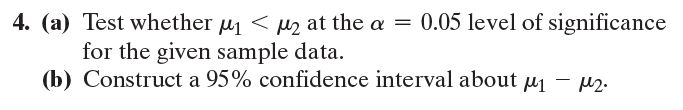×
Get Full Access to Statistics: Informed Decisions Using Data - 5 Edition - Chapter 11.3 - Problem 4
Get Full Access to Statistics: Informed Decisions Using Data - 5 Edition - Chapter 11.3 - Problem 4

×

# ?In Problems 1–6, assume that the populations are normally distributed. 4. (a) Test whether m1 6 m2 at the a = 0.05 level of significance for theISBN: 9780134133539 240

## Solution for problem 4 Chapter 11.3

Statistics: Informed Decisions Using Data | 5th Edition

• Textbook Solutions
• 2901 Step-by-step solutions solved by professors and subject experts
• Get 24/7 help from StudySoup virtual teaching assistantsStatistics: Informed Decisions Using Data | 5th Edition

4 5 1 382 Reviews
27
1
Problem 4

In Problems 1–6, assume that the populations are normally distributed.

4. (a) Test whether m1 6 m2 at the a = 0.05 level of significance for the given sample data.

(b) Construct a 95% confidence interval about m1 - m2.Step-by-Step Solution:

Step 1 of 5) In Problems 1–6, assume that the populations are normally distributed. 4. (a) Test whether m1 6 m2 at the a = 0.05 level of significance for the given sample data. (b) Construct a 95% confidence interval about m1 - m2. Because the P-value, 0.002, is less than the level of significance, a, we reject the null hypothesis. There is sufficient evidence to indicate that at least one population mean shear bond strength is different from the others. Whenever performing ANOVA, it is a good idea to present visual evidence that supports the conclusions of the test. Side-by-side boxplots are a great way to help visually reinforce the results of the ANOVA procedure. Figure 6 shows the side-by-side boxplots of the data presented in Table 1. The boxplots support the ANOVA results from Example 3. If we were conducting this ANOVA test by hand using the Classical Method, we would compare the F-test statistic with a critical F-value.

Step 2 of 2

## Discover and learn what students are asking

Calculus: Early Transcendental Functions : Basic Differentiation Rules and Rates of Change
?Finding a Value In Exercises 65–70, find k such that the line is tangent to the graph of the function. Function

Calculus: Early Transcendental Functions : Introduction to Functions of Several Variables
?In Exercises 19 - 30, find the domain and range of the function. $$f(x, y)=x^{2}+y^{2}$$

Calculus: Early Transcendental Functions : Iterated Integrals and Area in the Plane
?In Exercises 1 - 10, evaluate the integral. $$\int_{0}^{\sqrt{4-x^{2}}} x^{2} y d y$$

Chemistry: The Central Science : Introduction:Matter, Energy, and Measurement
?Which of the following diagrams represents a chemical change? [Section 1.3]

Statistics: Informed Decisions Using Data : Data Collection
?In Problems 1–5, provide a definition using your own words. 4. Observational study

Statistics: Informed Decisions Using Data : Properties of the Normal Distribution
?In Problems 29 and 30, draw a normal curve and label the mean and inflection points. ? = 30 and ? = 10

Unlock Textbook Solution